American Journal of Physics and Applications
Volume 4, Issue 1, January 2016, Pages: 12-19

Review Article

Atomic Data and Laser Transitions in as - Like Gallium

Amal Ibrahim Refaie1, Mohammed Nour El-Din2, Lamia Mohammed Ahmed2, Sami Allam1

1Physics Department, Faculty of Science, Cairo University, Cairo, Egypt

2Physics Department, Faculty of Science, Benha University, Benha, Egypt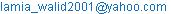(L. M. Ahmed)

Amal Ibrahim Refaie, Mohammed Nour El-Din, Lamia Mohammed Ahmed, Sami Allam. Atomic Data and Laser Transitions in as - Like Gallium. American Journal of Physics and Applications. Vol.4, No. 1, 2016, pp. 12-19. doi: 10.11648/j.ajpa.20160401.13

Abstract: Fine structure calculations of the energy levels, the wavelengths, the oscillator strengths, log gf and the transition probabilities for transitions among the terms belonging to 1s2 2s2 2p6 3s2 3p6 3d104s2ns, n=5-6, 1s2 2s2 2p6 3s2 3p6 3d104s2np, n=4-6, 1s2 2s2 2p6 3s2 3p6 3d104s2nd, n=4-6 and1s2 2s2 2p6 3s2 3p6 3d104s2nf, n=4-6 configurations of As (III) have been calculated using configurations interaction Cowan atomic structure code. Our calculated values for the above mentioned quantities have been compared with the corresponding experimental data and other theoretical calculations where a satisfactory agreement is found. We also report on some unpublished values for energy levels, oscillator strengths and transition probabilities for As like gallium. These atomic data are taken as the basis for studying laser transitions between levels of As(III). Excitation rate coefficients of As like gallium are calculated using the analytical formulas of Vriens and Smeets (1980) and with considering using the collisional radiative model code CRMO of Allam (2006). A simple modification to these formulas has been included by introducing effective quantum numbers. The energy values, the radiative data and rate coefficients are then used to calculate the population densities by solving the coupled rate equations. Among these calculations positive gain coefficients are found at three selected values of electron temperature, namely 7.087 eV, 14.147 eV and 21.261 eV which are displayed as a function of the electron impact density.

Keywords: Energy Levels, the Average Center of Mass Energy (Eav), the Spin-Orbit Interaction (x), Nist, the Oscillator Strength (ƒ), Rate Coefficients, Level Population, Maximum Gain Coefficient(αmax)

1. Introduction

Spectroscopic data of Gallium [1, 2] and Gallium-like ions [3-5] may be of interest for plasma investigations in controlled thermonuclear fusion experiments. Recently, multicharged ion spectra of the Gallium isoelectronic sequence (Z˃35) have been analyzed. In particular, spectra of the Rb(VII) ion emitted from sparks and laser-produced plasmas have been investigated . At the same time there are extensive experimental and theoretical spectroscopic studies for the Gallium like ions. The first six elements of the gallium sequence have been calculated using the multiconfiguration optimized potential model .

Gallium isoelectronic sequence has been studied for some transitions using the configuration interaction approach . Multiconfiguration Dirac-Fock calculations on multi-valence-electron systems have been also performed for Ga-like ions . In addition, spectra of the gallium-like ions excited by low-inductance sparks and laser-produced plasmas were observed . Oscillator strengths for excitations of As I-III are calculated using a semiemprical analytic independent-particle-model . Also the energy levels and observed spectral lines of Krypton, Kr I through Kr XXXVI have been studied .

The configuration interaction (CI) approach has been used to account for electron correlation effects. To visualize these effects we also include some single configuration results. Two self-consistent-field methods are used to generate orbitals required to construct a multiconfiguration wave function. One of them is the Hartree-Fock method with taking into account the relativistic corrections (HFR) and the other one is also Hartree Fock method but without considering the relativistic corrections.

The level population can be calculated by solving the coupled rate equations for the various levels in As (III) [13-17]. The electron collisional excitation rate coefficients are calculated according to the analytical formulas of Vriens and Smeets . Inversion factors are calculated after electron collisional pumping and the gain coefficients for those transitions that have positive population inversion have evaluated . Fine structure energy levels, wavelengths, log gf, oscillator strengths, and transition probabilities needed in calculations have been calculated using Cowan atomic structure code with relativistic corrections [20-22] for transitions among the terms belonging to 1s2 2s2 2p6 3s2 3p6 3d10ns, n=5-6, 1s2 2s2 2p6 3s2 3p6 3d10np, n=4-6, 1s2 2s2 2p6 3s2 3p6 3d10nd, n=4-6 and1s2 2s2 2p6 3s2 3p6 3d10nf, n=4-6 configurations of As like gallium.

It is found that COWAN code in addition to parametric fit approach is a useful tool for heavy many electron systems where intravelence electron correlation plays an essential role. The purpose of this paper is to report new calculations involving higher energy levels, wavelengths, oscillator strengths and transition probabilities that are not calculated before beside the precalculated data to indicate the reliabilities of the new ones by making comparisons with the available theoretical and experimental data.

2. Method of Calculations

Theoretical treatment of an atom containing N electrons requires first of all knowledge of a suitable Hamiltonian operator. An appropriate operator may be obtained by summing the one electron operator over all N electrons, and adding a term for electrostatic coulomb interactions among electrons 

H = Hkin + Helec-nucl + Helec- elec + HS-O =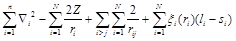(1)

where ri =is the distance of the ith electron from the nucleus,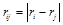is the distance between the ith and jth electron, and x(ri) is a radial proportional factor. It should be noticed that in a multi-electron atom, other magnetic interactions may be considered such as: orbital-orbital, spin-spin, and spin-other orbit interactions. But these interactions are usually much less important than the spin-orbital interaction, thus they can be neglected. Each electron in a many electron atom is considered to move in a central potential independently of the others , at least in the first approximation. The ith electron may then be described by the function Ψα(i) which is given in terms of a product of radial, spherical harmonic and spin wave functions:-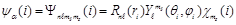(2)

where Rnℓ (ri) is the radial function,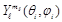is the spherical harmonic function,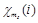is the spin function, n is the principal quantum number, m is the z-component of the electron orbital angular momentum quantum number, and ms is the z-component of the electron spin quantum number.

For N-electrons, the Pauli principle requires that each set of quantum numbers: a, b, g, …….n should be different from one electron to other. This means that a simple N-electron wave function will consist of a direct product of single-electron wave functions: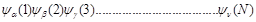The Pauli principle can be included in a more general symmetry principle, produced by Heisenberg and Dirac. Slater showed that this requirement could be satisfied by a normalized determinatal product wave function of the form:-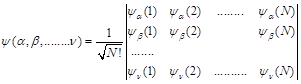(3)

In practice the linear combinations of such determinant functions for single configurations are formed which are angular eigenstates in an appropriate coupling scheme and these form the basis states for the multi-configuration expansions.

The matrix elements of the Hamiltonian between determinant functions can be reduced to one and two electron terms so that for states B and B′ [21, 22]: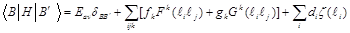(4)

where Eav is the central field energy. Furthermore, modest ad hoc scaling adjustments are applied to the F, G, and d to allow for configurations omitted from the multi-configuration expansion. The wave functions obtained from equation (3) are used to calculate the excitation energies of the fine structure levels, the oscillator strengths and the transition probabilities. The calculations are carried out in intermediate coupling scheme. A choice of nomenclatures is possible in seeking to assign angular quantum numbers as identifiers of the intermediate coupling numerical Eigen states. Since only parity and total angular momentum observables commute with the Hamiltonian, other quantum number assignments are approximated one. In these assignments we are led by angular momentum coupling schemes appropriate to the dominant parts of the Hamiltonian. One of them is known as the LS-scheme when the electrostatic terms dominate and the other is known as the jj-scheme when relativistic terms dominate. The code identifies the energy Eigen states by dominant component in its expansion in a particular basis.

The radial function is obtained as the solution of the following equation [21, 22]: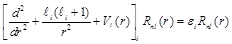The solution of the above Schrödinger equation (the radial wave function Rnl(r) is analogous to the behavior of legender Pnl(r) for which we have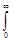Pnℓ (r) Pn`ℓ (r) dr = dnn`; ℓ +1 < n` £ n

where Vi is the central potential seen by the ith electron and εi is the single particle energy. An optimized potential is obtained variationally. It is noticeable that the present code uses the Relativistic Hartree Fock method of Cowan .

2.1. Spontaneous Transitions Between Degenerate Levels

The radiative decay rate between degenerate levels p and q is given by :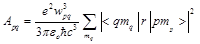(5)

where mp and mq are the magnetic orbital quantum numbers of the involved levels, e is the electron charge, c is the speed of light,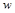is the angular frequency of the transition photon, and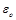is the vacuum permittivity.

The decay rate can be made more symmetrical by introducing an additional summation over mp and dividing by the statistical weight gp=2jp +1 of the upper level: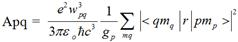(6)

The radiative life time tp of an excited atomic state p is related to the atomic transition probability Apq by: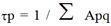(7)

where, the sum is extended over all the lower states which can be reached from the upper state by radiative decay.

2.2. Oscillator Strengths

The oscillator strength or f-value describes what fraction of the energy of the classical oscillator should be ascribed to a given transition . For transitions from an upper level p to a lower level q, the emission f-value, fpq is given by the following relation.

Apq = -3fpq g                   (8)

where: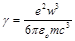is defined as the classical transition probability. While for an upward transition from level q to level p, the absorption f-value, fqp, is defined by:

gqfqp = - gp fpq                (9)

The explicit expressions for the transition probability Apq in terms of the emission and the absorption oscillator strengths are given by the following relation: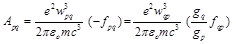(10)

2.3. Calculation of Rate Coefficients

The collisional excitation is the mechanism used routinely in all running lasers to excite an electron from the fundamental level to the upper laser level (i.e. to pump the laser). The collisional excitation is accomplished by high temperature free electrons in plasma that collide with ions. Vriens and Smeets  (1980) construct analytic semiempirical formula for the excitation rate of coefficient from lower level p to upper level q for hydrogenic atoms. This formula is given by the following:-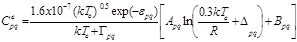(11)

Where, both electron temperature, kTe and the Rydberg energy, R are in eV, Epq = Eq-Ep is the energy difference between the two levels and fpq is the absorption oscillator strength. The values of the parameters Gpq, Dpq, Apq and Bpq are obtained in detail from ref. .

For a hydrogen atom p and q are the principal quantum numbers. For atoms or ions with a single electron outside closed shell the principle quantum numbers are replaced by effective quantum numbers in the above mentioned equations, i.e.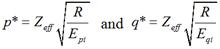(12)

where Epi and Eqi are the ionization energies of the lower and upper levels respectively and Zeff is the effective nuclear charge. Excitation rate coefficient can be calculated with the aid of a computer program of Allam (CRMO) , which has been developed for collisional radiative models calculations.

2.4. Calculation of Level Population

The level population Nq are calculated by solving the coupled rate equations (26)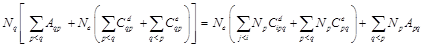(13)

where Nq and Np is the population of the level q and p, espectively, Aqp is the spontaneous decay rate from level q to level p, Ceqp and Cdpq are the electron collisional excitation and de-excitation rate coefficient, respectively, and, Ne is the electrons densities

2.5. Inversion Factor and Gain Coefficient

In high temperature plasma, Doppler broadening is expected to be the main source of line broadening. Under these conditions the gain coefficient is given by :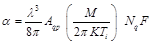(14)

where F is the inversion factor and it is given by: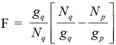(15)

where Nq/gq and Np/gp are the reduced populations of the upper and lower level respectively. When the quantity between parentheses is positive, i.e. (Nq/gq) > (Np/gp), there is population inversion between the two levels. This will lead to positive inversion factor (F) and positive gain coefficient, and hence, to a possible laser emission.

3. Results and Discussion

3.1. Energy Levels

In general, with one electron outside a closed shell, correlation in the core is negligible . The energy of the electronic configurations are obtained by adjusting the scalling parameters Eav and ζi (ri) that are shown in tables 1. The energy levels for As-like gallium have been tabulated in tables 2. Our calculations of energy levels have been compared with data relative to NIST From the comparison we found that all the calculated energy levels are almost the same to the values of Nist .

Table 1. Radial function parameters for Gallium-like ions in units of 1000 cm-1.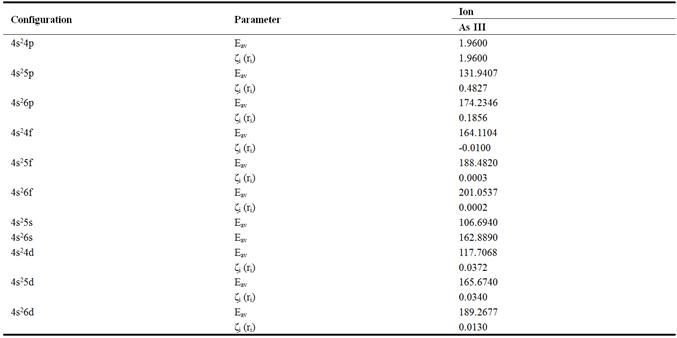Table 2. Energy levels in cm-1 for As III calculated by COWAN code in Comparison with Nist  experimental values.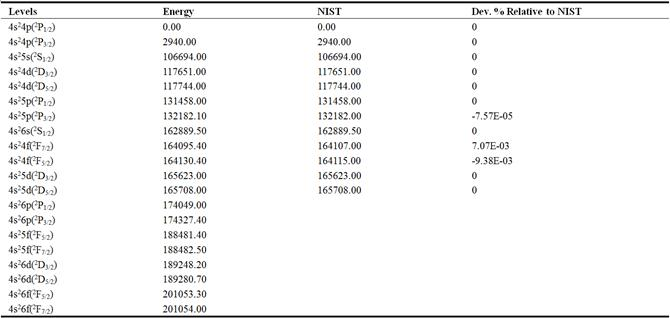It is clear, from table (2) that we have get a good agreement with the with Nist  experimental values.

3.2. Wavelengths, Oscillator Strengths and Transition Probabilities

In tables 3 we have tabulated our calculated wavelengths, λ (Å), the absorption oscillator strength, ƒ, log(Gƒ) and the radiative decay rates, A, for all allowed electric dipole transitions among As III.

Table 3. Wavelengths, log gf, oscillator strengths and radiative decay rates for As III.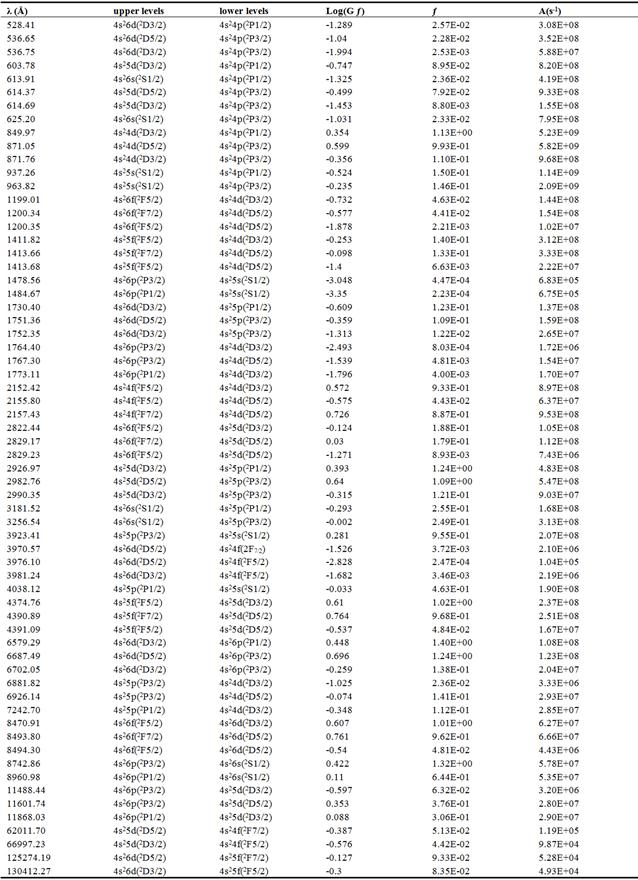3.3. Excitation Rate Coefficients

Excitation rate coefficients (cm3 sec-1) as a function of electron temperature (eV) for 4s24d(2D3/2)-4s25p(2P1/2), 4s24d(2D3/2)-4s25p(2P3/2) and 4s24d(2D5/2)-4s25p(2P3/2) transitions, in case of As(III), are given in Figure (1) only for transitions where population inversion occur.Fig. 1. Exitation rate coefficients of As(III).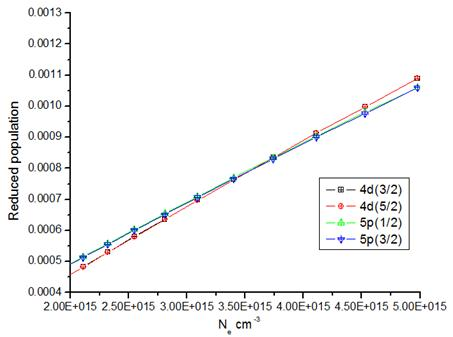Fig. 2. Reduced populations for selected levels of As(III) at electron temperature 21.261 eV.

3.4. Level Population

The level population for 4s24d(2D3/2), 4s24d(2D5/2), 4s25p(2P1/2) and 4s25p(2P3/2) levels are calculated at electron temperatures equal to 7.087 eV, 14.147 eV and 21.261 eV. The behavior of the reduced population is illustrated in figure (2) at electron temperatures equal to 21.261 eV.

The behavior of level populations of the various ions can be explained as follows: in fig (2), the 4s24d(2D3/2) and 4s24d(2D5/2) levels cannot decay fast to the 4s25p(2P1/2) and 4s25p(2P3/2) levels. So by time the population of 4s24d(2D3/2) and 4s24d(2D5/2) levels become greater than the population of 4s25p(2P1/2) and 4s25p(2P3/2) levels. Therefore the population inversions occur between these two levels. In general the behavior of population in finger (2) can be explained as follows: in the case of collisional pumping, at low densities, the reduced populations increase as functions of electron density. This is due to the increase in the collisional excitation rates with density [13-17]. At high electron density, where the collisional excitation rates exceed the radaitive decay rates, the reduced populations are independent of electron density and are approximately equal. The population inversion is largest where the electron collisional de-excitation rate for upper level is comparable to the radiative decay rate for this level approximately equal.

3.5. Gain Coefficient

As a result of population inversion there will be a positive gain in the laser medium. Equation (14) has been used to calculate the gain coefficient for the Doppler broadening of various transitions in As(III).

Our gain results for: 4s25p(2P1/2)- 4s24d(2D3/2), 4s25p(2P3/2)- 4s24d(2D3/2) and 4s25p(2P3/2)- 4s24d(2D5/2) transitions, as a function of the electron density for As (III) at electron temperatures 7.087 eV, 14.147 eV and 21.261 eV, are tabulated in table (4) which contains the parameters for the laser medium and the laser transition. That is to say the wavelength l (Å) of transition, the life time of the upper laser levels tq (ns), the lifetime of the lower laser level tp (ns), the plasma electron density Ne(cm-3), the plasma electron temperature Te(eV), and the maximum gain coefficient a(cm-1). The behavior of the laser gain as function of electron density at electron temperature 7.087 eV, 14.147 eV and 21.261 eV shown in figures (3 - 5).

Table 4. Parameters of the most intense laser transition in As(III).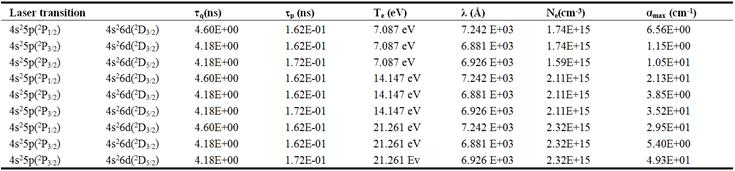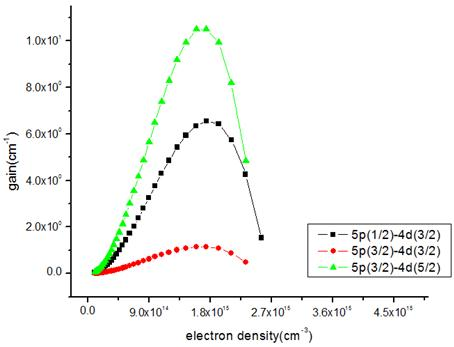Fig. 3. Gain coefficient of laser transitions via electron collisional excitation pumping at electron temperature equal to7.087 eV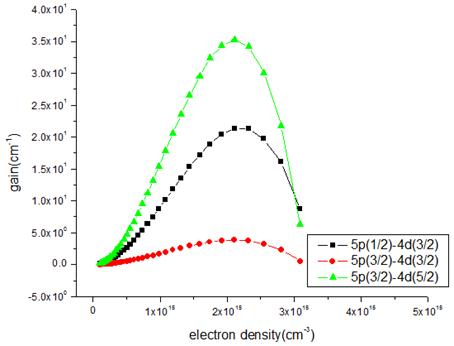Fig. 4. Gain coefficient of laser transitions via electron collisional excitation pumping at electron temperature equal to 14.147 eV.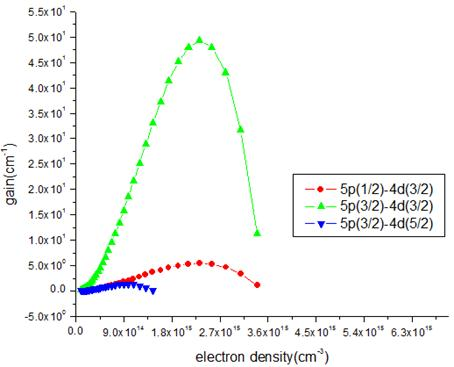Fig. 5. Gain coefficients of laser transitions via electron collisional excitation pumping at electron temperature equal to 21.261 eV.

The above three figures for gain coefficient show that the population inversions occur for several transitions in As (III). However, the largest gain has been existed for the 4s25p(2P3/2)- 4s26d(2D5/2) transition producing gain value of 4.93E+01 (cm-1) with wavelength of 6.926 E+03 (Å). Our calculations show, under favorable conditions, that a significant laser gain transitions can be achieved in As-like Ga. The behavior of the gain coefficients a (cm-1) as a function of electron density (cm-3) can be explained as follows: at low electron density, the gain increases approximately as the electron density increases. This means that the fractional populations increase linearly with the electron density due to the increase of the collisional excitation rate [30, 31]. The maximum gain occurs at about the density where collisional depopulation of the level becomes comparable to radiative decay. At (1015£ Ne£1016), the upper level population increases slower than Ne while the lower level populations continue to increase together with Ne and therefore, the inversion factor (F), begin to decrease and the gain coefficient decrease.

4. Conclusion

It is clear that the present calculations of energy levels and wavelengths show good agreement with the corresponding available both theoretical and experimental data. We have obtained some new unpublished energy levels, wavelengths and transition probabilities for these ions. These extensive and the more definitive results may be useful in thermonuclear fusion research and astrophysical applications.

The population of each level in As-like gallium is calculated and used to calculate the gain coefficient. The values of the maximum gain coefficients ranges from 1.15E+00 to 4.93E+01 (cm-1). Our calculations may give an attribute in the production of laser by collisional pumping under actual experimental conditions. In this case additional processes, such as radiative, dielectronic recombination, and perhaps resonances can significantly affect level populations which needs a future study.

References

1. D. Iablonskyi, K. Jänkälä, S. Urpelainen and M. Huttula, J. Phys. B: At. Mol. Opt. Phys.46, 175001 (2013).
2. Yu. M. Smirnov, Opt. Spectrosc. 110, 1–8 (2011).
3. J. E. Sansonetti, J. Phys. Chem. Ref. Data 41, 013102 (2012).
4. J. E. Sansonetti, J. Phys. Chem. Ref. Data 35, 301 (2006).
5. J. E. Sansonetti and W. C. Martin, J. Phys. Chem. Ref. Data 34, 1559 (2005).
6. E. Biémont, P. Quinet,J.Quant.spectrosc.Radiat.Transfer Vol.44, No.2,pp. 233-244, 1990.
7. K. Aashamar, T. M. Luke and J. D. Talman,J. Phys. B: At. Mol. Phys. 16 (1983) 2695-2708.
8. R. Marcinek and J. Migdalek,J. Phys. B: At. Mol. Opt. Phys. 26 (1993) 1403 1414.
9. F. Hu, J. Yag, C. Wang, L. Jing, S. Chen, G. Jiang, H. Liu, L. Hao; Phys. Rev. A 84, 042506 (2011).
10. Reader, J., Acquista, N. and Goldsmith, S., J. Opt. Soc. Am. B 3, 874 (1986).
11. P. S. Ganas, Astron. Astrophys., Suppl.Ser. 143, 491 (2000).
12. E. B. Saloman, J. Phys. Chem. Ref. Data 36, 215 (2007).
13. Feldman U, Bhatia A.K, Suckewer S; J. Appl. Phys. 5, 1983. p. 54.
14. Feldman U, Doschek G. A, and Seely J. F, Bhatia A. K; J. Appl. Phys. 58, 1985. p. 2909-2915.
15. Feldman U, Seely J. F and Doschek G. A; J. de Physique, C6, 1986.p.187.
16. Feldman U, Seely J. F and Doschek G. A, Bhatia A. K; J. Appl. Phys. 59, 1986. p. 3953.
17. Feldman U, Seely J. F and Bhatia A. K; J. Appl. Phys. 56, 1984. p. 2475.
18. Vriens L and Smeets A. H. M; Phys. Rev. A 22, 1980. p. 3.
19. Fowles R. Introduction to modern optics. Holt, Rinehart, and Winston, New York. 1968.
20. http://www.tcd.ie/Physics/People/Cormac.McGuinness/Cowan/.
21. Cowan R.D, The theory of atomic structure and spectra, University of California Press, Berkeley - Los Angeles-London, 1981.
22. Cowan R. D, J. Opt. Soc. Am.58, 1968. p. 808.
23. Sobelman "Introduction to the Theory of Atomic Spectra", international series of monographs in Natural Philosophy,Pergamon Press,Vol.40,(1979).
24. Alan Corney"Atomic And Laser Spectroscopy"Oxford Univesity Press(1977).
25. Allam S. H "CRMO-Collisional Radiative Model" computer code,Private communication.
26. Silfvast W.T, Green J.M, and Wood O.R. Phys. Rev. Lett. 35, 1975. p. 435.
27. Fowles R. Introduction to modern optics. Holt, Rinehart, and Winston, New York. 1968.
28. J.C.Slater, "Quantum Theory of Atomic Structure", Vols.I and II, McGraw-Hill, New York,(1960).
29. D. E. Kellcher, W. C. Martin., W. L. Wiese, J. Suger, J. R Fuher, K. Olsen, A. Musgrove, P. J. Mohr, J. Reader and G. R. Dalton, Phys. Scr. 83 (1999)158, http://physics.nist.gov/cgi-bin/atdata/main_asd.
30. John J. Brehm and William J. Mullin, "introduction to the structure of Matter", John Wiley & Sons, 1989.
31. Mahmoud Ahmad, Ahmed Abou El-Maaref, Essam Abdel-Wahab1, Sami Allam,American Journal of Optics and Photonics. Vol. 3, No. 1, 2015, pp. 17-23.

 Contents 1. 2. 2.1. 2.2. 2.3. 2.4. 2.5. 3. 3.1. 3.2. 3.3. 3.4. 3.5. 4.
Article ToolsAbstractPDF(965K)